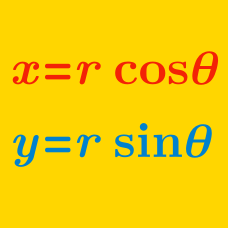Algebra

# Converting Polar Coordinates to Cartesian

The point $\left(12, \frac{3\pi}{4} \right)$ in polar coordinates can be expressed as $(a, b)$ in Cartesian coordinates, where $a$ and $b$ are real numbers. What is the value of $-ab?$

Suppose that $(a, \frac{\pi}{6})$ in polar coordinates represents the same point as $(b, 7)$ in Cartesian coordinates. What is the value of $a^2+b^2$?

$P=(24, \frac{\pi}{3})$ in polar coordinates can be expressed as $P=(a, b)$ in Cartesian coordinates. What is the value of $a+b^2$?

If point $P$ is given in polar coordinates as $P=\left(-10, \frac{3\pi}{4}\right),$ what are the Cartesian coordinates of $P?$

In polar coordinates, the graph of $r = 46 \sin(\theta)$ is a circle. What is the radius of this circle?

×